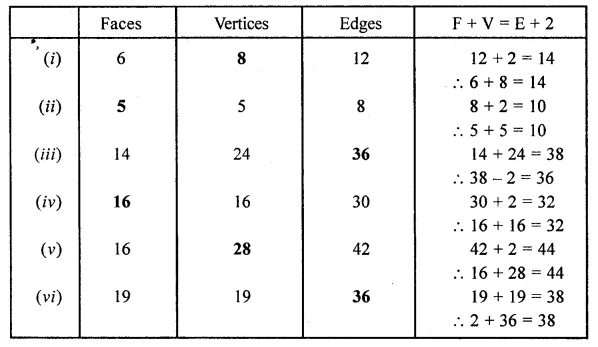# ML Aggarwal Class 8 Solutions for ICSE Maths Chapter 17 Visualising Solid Shapes Ex 17.2

## ML Aggarwal Class 8 Solutions for ICSE Maths Chapter 17 Visualising Solid Shapes Ex 17.2

Question 1.
Can a polyhedron have for its faces
(i) 3 triangles?
(ii) 4 triangles?
(iii) a square and four triangles?
Solution: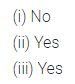Question 2.
Which are prisms among the following?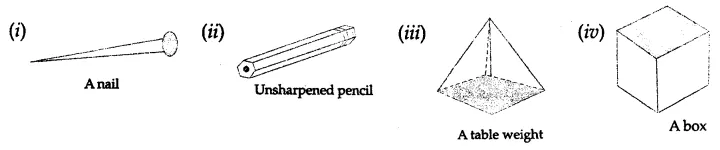Solution:Question 3.
Verify Euler’s formula for these solids: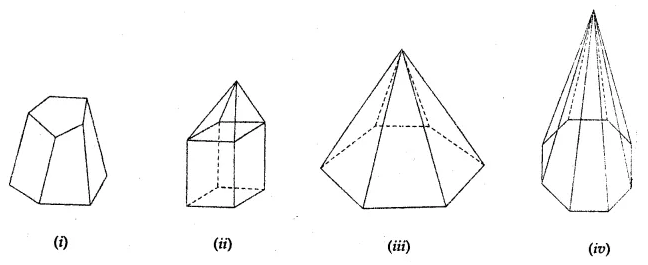Solution:Question 4.
Can a polyhedron have 15 faces, 30 edges and 20 vertices?
Solution: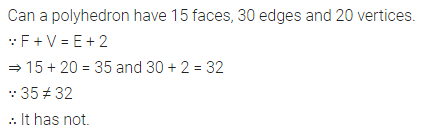Question 5.
If a polyhedron has 8 faces and 8 vertices, find the number of edges.
Solution:Question 6.
If a polyhedron has 7 faces and 10 vertices, find the number of edges.
Solution:Question 7.
Write the number of faces, vertices and edges in
(i) an octagonal prism
(ii) decagonal pyramid.
Solution: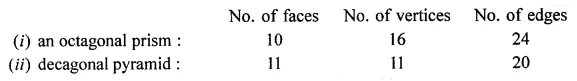Question 8.
Using Euler’s formula, complete the following table:Solution: